Open In App

# Multiprocessing in Python | Set 2 (Communication between processes)

Multiprocessing in Python | Set 1
These articles discusses the concept of data sharing and message passing between processes while using multiprocessing module in Python.
In multiprocessing, any newly created process will do following:

• run independently
• have their own memory space.

Consider the program below to understand this concept:

 `import` `multiprocessing`` ` `# empty list with global scope``result ``=` `[]`` ` `def` `square_list(mylist):``    ``"""``    ``function to square a given list``    ``"""``    ``global` `result``    ``# append squares of mylist to global list result``    ``for` `num ``in` `mylist:``        ``result.append(num ``*` `num)``    ``# print global list result``    ``print``(``"Result(in process p1): {}"``.``format``(result))`` ` `if` `__name__ ``=``=` `"__main__"``:``    ``# input list``    ``mylist ``=` `[``1``,``2``,``3``,``4``]`` ` `    ``# creating new process``    ``p1 ``=` `multiprocessing.Process(target``=``square_list, args``=``(mylist,))``    ``# starting process``    ``p1.start()``    ``# wait until process is finished``    ``p1.join()`` ` `    ``# print global result list``    ``print``(``"Result(in main program): {}"``.``format``(result))`

```Result(in process p1): [1, 4, 9, 16]
Result(in main program): []
```

In above example, we try to print contents of global list result at two places:

• In square_list function. Since, this function is called by process p1, result list is changed in memory space of process p1 only.
• After the completion of process p1 in main program. Since main program is run by a different process, its memory space still contains the empty result list.

Diagram shown below clears this concept: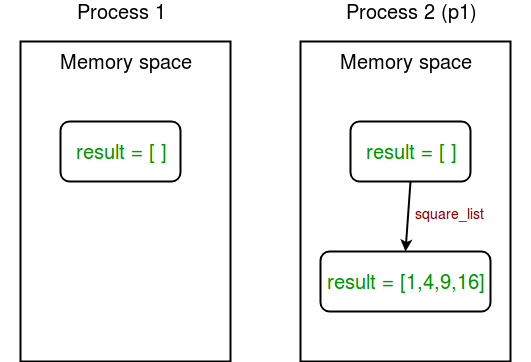Sharing data between processes

1. Shared memory : multiprocessing module provides Array and Value objects to share data between processes.
• Array: a ctypes array allocated from shared memory.
• Value: a ctypes object allocated from shared memory.

Given below is a simple example showing use of Array and Value for sharing data between processes.

 `import` `multiprocessing`` ` `def` `square_list(mylist, result, square_sum):``    ``"""``    ``function to square a given list``    ``"""``    ``# append squares of mylist to result array``    ``for` `idx, num ``in` `enumerate``(mylist):``        ``result[idx] ``=` `num ``*` `num`` ` `    ``# square_sum value``    ``square_sum.value ``=` `sum``(result)`` ` `    ``# print result Array``    ``print``(``"Result(in process p1): {}"``.``format``(result[:]))`` ` `    ``# print square_sum Value``    ``print``(``"Sum of squares(in process p1): {}"``.``format``(square_sum.value))`` ` `if` `__name__ ``=``=` `"__main__"``:``    ``# input list``    ``mylist ``=` `[``1``,``2``,``3``,``4``]`` ` `    ``# creating Array of int data type with space for 4 integers``    ``result ``=` `multiprocessing.Array(``'i'``, ``4``)`` ` `    ``# creating Value of int data type``    ``square_sum ``=` `multiprocessing.Value(``'i'``)`` ` `    ``# creating new process``    ``p1 ``=` `multiprocessing.Process(target``=``square_list, args``=``(mylist, result, square_sum))`` ` `    ``# starting process``    ``p1.start()`` ` `    ``# wait until the process is finished``    ``p1.join()`` ` `    ``# print result array``    ``print``(``"Result(in main program): {}"``.``format``(result[:]))`` ` `    ``# print square_sum Value``    ``print``(``"Sum of squares(in main program): {}"``.``format``(square_sum.value))`

```Result(in process p1): [1, 4, 9, 16]
Sum of squares(in process p1): 30
Result(in main program): [1, 4, 9, 16]
Sum of squares(in main program): 30
```

Let us try to understand the above code line by line:

• First of all, we create an Array result like this:
``````  result = multiprocessing.Array('i', 4)
``````
• First argument is the data type. ‘i’ stands for integer whereas ‘d’ stands for float data type.
• Second argument is the size of array. Here, we create an array of 4 elements.

Similarly, we create a Value square_sum like this:

``````  square_sum = multiprocessing.Value('i')
``````

Here, we only need to specify data type. The value can be given an initial value(say 10) like this:

``````  square_sum = multiprocessing.Value('i', 10)
``````
• Secondly, we pass result and square_sum as arguments while creating Process object.
``````  p1 = multiprocessing.Process(target=square_list, args=(mylist, result, square_sum))
``````
• result array elements are given a value by specifying index of array element.
``````  for idx, num in enumerate(mylist):
result[idx] = num * num
``````

square_sum is given a value by using its value attribute:

``````  square_sum.value = sum(result)
``````
• In order to print result array elements, we use result[:] to print complete array.
``````  print("Result(in process p1): {}".format(result[:]))
``````

Value of square_sum is simply printed as:

``````  print("Sum of squares(in process p1): {}".format(square_sum.value))
``````

Here is a diagram depicting how processes share Array and Value object: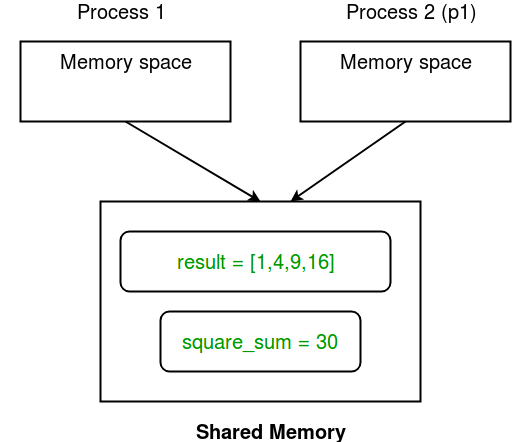2. Server process : Whenever a python program starts, a server process is also started. From there on, whenever a new process is needed, the parent process connects to the server and requests it to fork a new process.
A server process can hold Python objects and allows other processes to manipulate them using proxies.
multiprocessing module provides a Manager class which controls a server process. Hence, managers provide a way to create data that can be shared between different processes.

Server process managers are more flexible than using shared memory objects because they can be made to support arbitrary object types like lists, dictionaries, Queue, Value, Array, etc. Also, a single manager can be shared by processes on different computers over a network. They are, however, slower than using shared memory.

Consider the example given below:

 `import` `multiprocessing`` ` `def` `print_records(records):``    ``"""``    ``function to print record(tuples) in records(list)``    ``"""``    ``for` `record ``in` `records:``        ``print``(``"Name: {0}\nScore: {1}\n"``.``format``(record[``0``], record[``1``]))`` ` `def` `insert_record(record, records):``    ``"""``    ``function to add a new record to records(list)``    ``"""``    ``records.append(record)``    ``print``(``"New record added!\n"``)`` ` `if` `__name__ ``=``=` `'__main__'``:``    ``with multiprocessing.Manager() as manager:``        ``# creating a list in server process memory``        ``records ``=` `manager.``list``([(``'Sam'``, ``10``), (``'Adam'``, ``9``), (``'Kevin'``,``9``)])``        ``# new record to be inserted in records``        ``new_record ``=` `(``'Jeff'``, ``8``)`` ` `        ``# creating new processes``        ``p1 ``=` `multiprocessing.Process(target``=``insert_record, args``=``(new_record, records))``        ``p2 ``=` `multiprocessing.Process(target``=``print_records, args``=``(records,))`` ` `        ``# running process p1 to insert new record``        ``p1.start()``        ``p1.join()`` ` `        ``# running process p2 to print records``        ``p2.start()``        ``p2.join()`

```New record added!

Name: Sam
Score: 10

Score: 9

Name: Kevin
Score: 9

Name: Jeff
Score: 8
```

Let us try to understand above piece of code:

• First of all, we create a manager object using:
``````  with multiprocessing.Manager() as manager:
``````

All the lines under with statement block are under the scope of manager object.

• Then, we create a list records in server process memory using:
``````  records = manager.list([('Sam', 10), ('Adam', 9), ('Kevin',9)])
``````

Similarly, you can create a dictionary as manager.dict method.

• Finally, we create to processes p1 (to insert a new record in records list) and p2 (to print records) and run them while passing records as one of the arguments.

The concept of server process is depicted in the diagram shown below: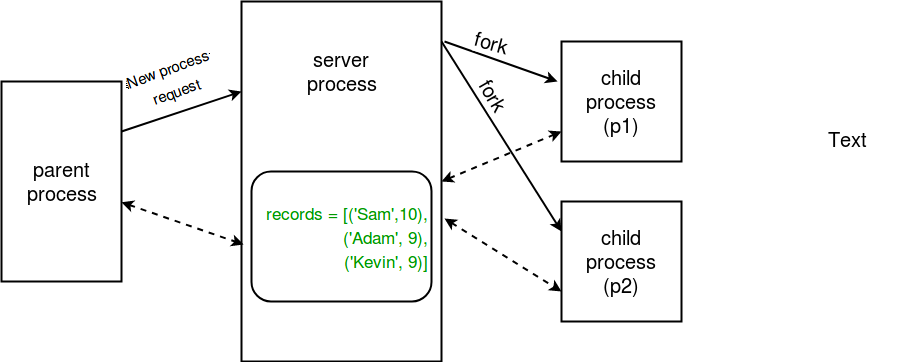Communication between processes

Effective use of multiple processes usually requires some communication between them, so that work can be divided and results can be aggregated.
multiprocessing supports two types of communication channel between processes:

• Queue
• Pipe
1. Queue : A simple way to communicate between process with multiprocessing is to use a Queue to pass messages back and forth. Any Python object can pass through a Queue.
Note: The multiprocessing.Queue class is a near clone of queue.Queue.
Consider the example program given below:

 `import` `multiprocessing`` ` `def` `square_list(mylist, q):``    ``"""``    ``function to square a given list``    ``"""``    ``# append squares of mylist to queue``    ``for` `num ``in` `mylist:``        ``q.put(num ``*` `num)`` ` `def` `print_queue(q):``    ``"""``    ``function to print queue elements``    ``"""``    ``print``(``"Queue elements:"``)``    ``while` `not` `q.empty():``        ``print``(q.get())``    ``print``(``"Queue is now empty!"``)`` ` `if` `__name__ ``=``=` `"__main__"``:``    ``# input list``    ``mylist ``=` `[``1``,``2``,``3``,``4``]`` ` `    ``# creating multiprocessing Queue``    ``q ``=` `multiprocessing.Queue()`` ` `    ``# creating new processes``    ``p1 ``=` `multiprocessing.Process(target``=``square_list, args``=``(mylist, q))``    ``p2 ``=` `multiprocessing.Process(target``=``print_queue, args``=``(q,))`` ` `    ``# running process p1 to square list``    ``p1.start()``    ``p1.join()`` ` `    ``# running process p2 to get queue elements``    ``p2.start()``    ``p2.join()`

```Queue elements:
1
4
9
16
Queue is now empty!
```

Let us try to understand the above code step by step:

• Firstly, we create a multiprocessing Queue using:
``````  q = multiprocessing.Queue()
``````
• Then we pass empty queue q to square_list function through process p1. Elements are inserted to queue using put method.
``````  q.put(num * num)
``````
• In order to print queue elements, we use get method until queue is not empty.
``````  while not q.empty():
print(q.get())
``````

Given below is a simple diagram depicting the operations on queue: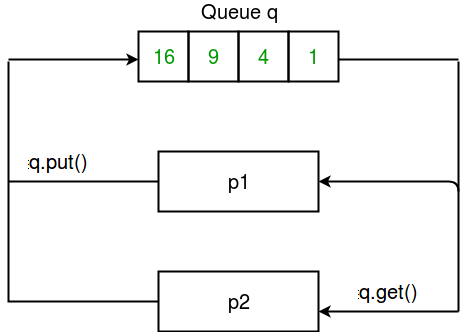2. Pipes : A pipe can have only two endpoints. Hence, it is preferred over queue when only two-way communication is required.

multiprocessing module provides Pipe() function which returns a pair of connection objects connected by a pipe. The two connection objects returned by Pipe() represent the two ends of the pipe. Each connection object has send() and recv() methods (among others).
Consider the program given below:

 `import` `multiprocessing`` ` `def` `sender(conn, msgs):``    ``"""``    ``function to send messages to other end of pipe``    ``"""``    ``for` `msg ``in` `msgs:``        ``conn.send(msg)``        ``print``(``"Sent the message: {}"``.``format``(msg))``    ``conn.close()`` ` `def` `receiver(conn):``    ``"""``    ``function to print the messages received from other``    ``end of pipe``    ``"""``    ``while` `1``:``        ``msg ``=` `conn.recv()``        ``if` `msg ``=``=` `"END"``:``            ``break``        ``print``(``"Received the message: {}"``.``format``(msg))`` ` `if` `__name__ ``=``=` `"__main__"``:``    ``# messages to be sent``    ``msgs ``=` `[``"hello"``, ``"hey"``, ``"hru?"``, ``"END"``]`` ` `    ``# creating a pipe``    ``parent_conn, child_conn ``=` `multiprocessing.Pipe()`` ` `    ``# creating new processes``    ``p1 ``=` `multiprocessing.Process(target``=``sender, args``=``(parent_conn,msgs))``    ``p2 ``=` `multiprocessing.Process(target``=``receiver, args``=``(child_conn,))`` ` `    ``# running processes``    ``p1.start()``    ``p2.start()`` ` `    ``# wait until processes finish``    ``p1.join()``    ``p2.join()`

```Sent the message: hello
Sent the message: hey
Sent the message: hru?
Sent the message: END
```

Let us try to understand above code:

• A pipe was created simply using:
``````  parent_conn, child_conn = multiprocessing.Pipe()
``````

The function returned two connection objects for the two ends of the pipe.

• Message is sent from one end of pipe to another using send method.
``````  conn.send(msg)
``````
• To receive any messages at one end of a pipe, we use recv method.
``````  msg = conn.recv()
``````
• In above program, we send a list of messages from one end to another. At the other end, we read messages until we receive “END” message.

Consider the diagram given below which shows the relation b/w pipe and processes: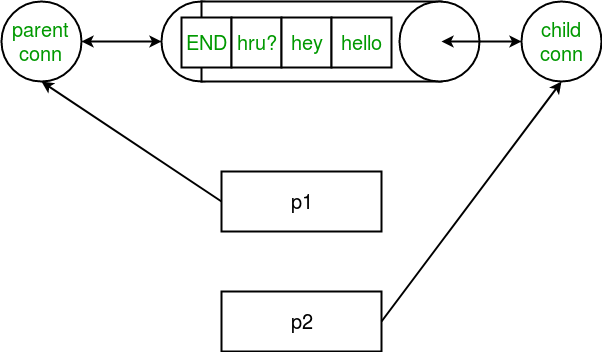Note: Data in a pipe may become corrupted if two processes (or threads) try to read from or write to the same end of the pipe at the same time. Of course, there is no risk of corruption from processes using different ends of the pipe at the same time. Also note that Queues do proper synchronization between processes, at the expense of more complexity. Hence, queues are said to be thread and process safe!

Next:

This article is contributed by Nikhil Kumar. If you like GeeksforGeeks and would like to contribute, you can also write an article using write.geeksforgeeks.org or mail your article to review-team@geeksforgeeks.org. See your article appearing on the GeeksforGeeks main page and help other Geeks.